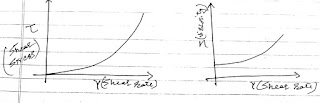## Monday, 5 October 2015

### Basics of Rheology of pharmaceutics for GPAT preparation

Study of the flow of materials that behave that behave in an interesting or unusual manner is known as Rheology.

Consider the following situation:Newtonian flow of fluid

Shear stress is given by, τ = Force/Area
Shear Rate, γ = Small change in velocity (dv)/Small height (dh)

Newton's law of flow:  According to Newton's law of fluid, Shear stress is directly proportional to the shear rate.

By equation,

τ α γ       or      τ = ηγGraphical representation of Newtonian fluids

• Here, proportionality sign η represents the dynamic velocity having unit Pascal. SI unit is Poise.
• Kinematic Viscosity is given as, Dynamic Viscosity(η)/ Density of the fluid (ρ). Units are meter square per second or stokes.
• Fluids obeying Newton's law of fluid are known as Newtonian fluids. e.g. water, Benzene, Mineral oil, sugar solution etc.

Non-Newtonian systems: Fluids which do not obey Newton's law of flow.

a) Pseudoplastic behaviour: In this fluid, viscosity is inversely proportional  to the shear rate. In other words, as the rate of shear is increased viscosity of these fluid decreases. Expressions for these fluid is; τ = Kγn, where n is less than 1.Pseudoplastic fluid

e.g. Suspensions, Dispersion, Creams, Lotions, Gels etc.

b) Dilatant: In this fluid, viscosity is directly proportional to the shear rate. In other words, as the rate of shear is increased viscosity of these fluid decreases. Expressions for these fluid is; τ = Kγn, where n is greater than 1.
e.g. Surfactant solution, Corn starch dispersion, Wet sand etc.Dilatant Flow

c) Plastic fluid (Bingham bodies): These types of fluid do not show any shear rate initially after applying shear stress. After a certain value of shear stress they start behaving like Newtonian fluid. Toothpaste is the classic example of plastic fluid.Bingham bodies (Plastic flow)

• Yield point(τ0): It is the max value of shear stress (τ) at which shear stress remains 0 in plastic bodies.
d) Thixotropy: In this type of behavior, viscosity of the fluid is decreased with shear stress, after removal of the stress fluid returns to the normal. Such systems are also known as Gel-Sol-Gel transformation.Thixotropy

e.g. Paints, Ketchup, Pastes etc.

e) Rheopexy: This is totally opposite to the thixotropy and therefore also known as negative thixotropy. In this type of behavior, viscosity of the fluid is increased with shear stress, after removal of the stress fluid returns to the normal. Such systems are also known as Sol-Gel-Sol transformation.Rheopexy

e.g. Latex dispersion.

This is an initiative to help our pharma community through spreading the knowledge. We are here committed to provide you high quality study material free of cost. If you also want to be the part of this initiative just like and share our facebook page so that more and more people can be benefited. Here is the link of our FB community: GPATCRACKERS